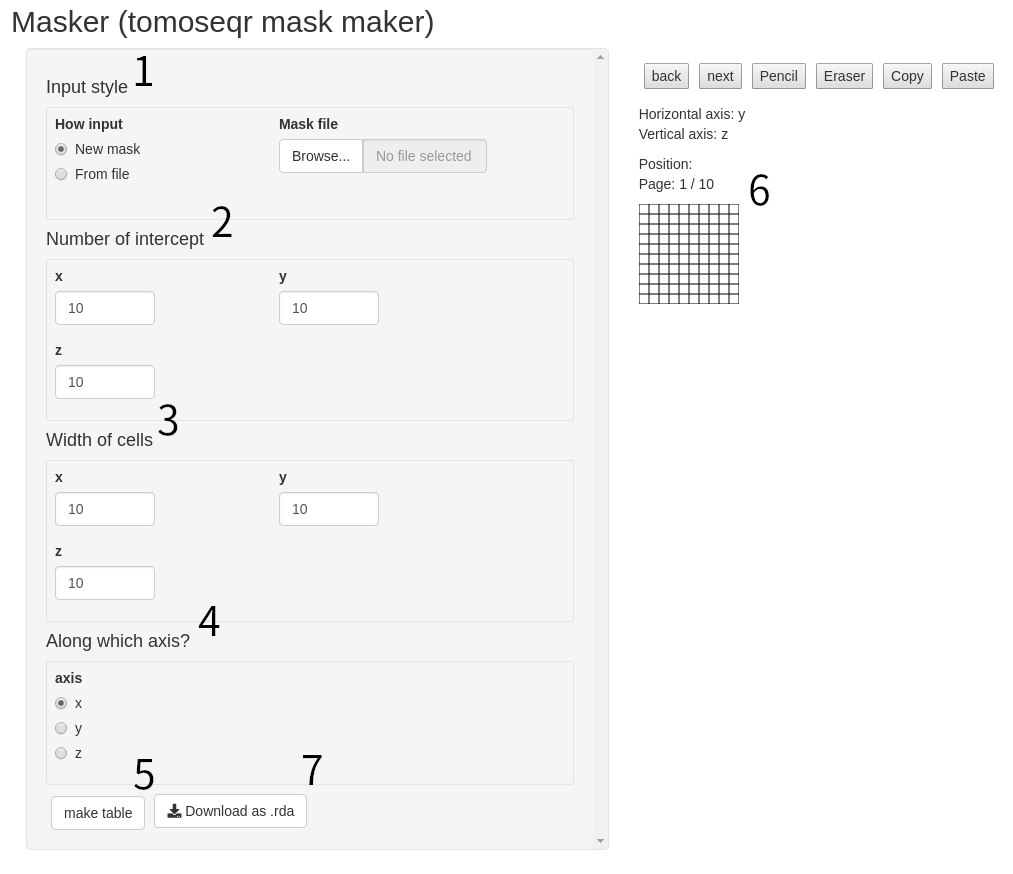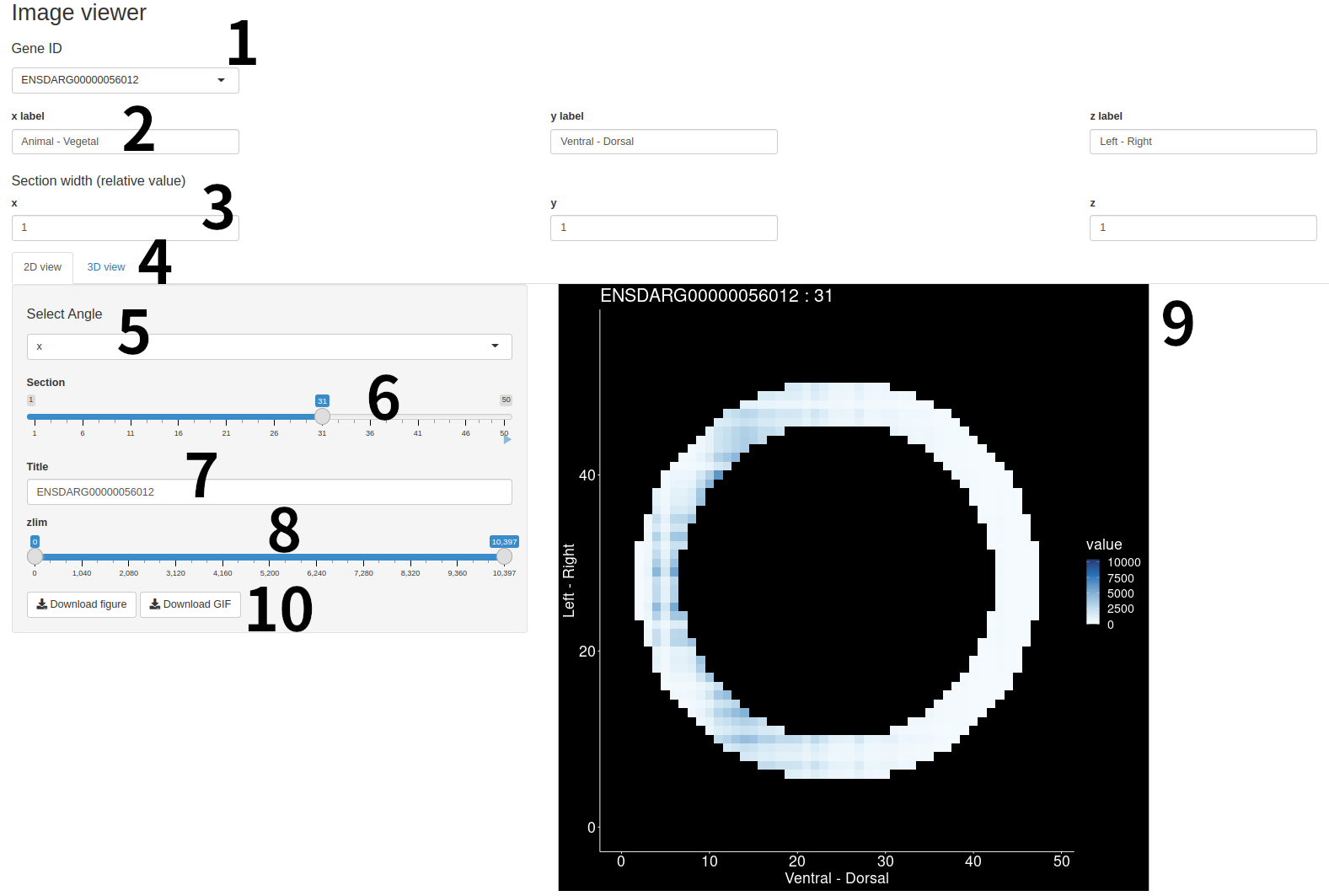# 1 Introduction

Tomo-seq is a genome-wide RNA tomography method that combines combining high-throughput RNA sequencing with cryosectioning for spatially resolved transcriptomics. In 2014, Junker et al. published a method for reconstructing 3D gene expression patterns from tomoseq data (iterative proportional fitting (Fienberg, 1970)). tomoseqr is a package to run this method in R. tomoseqr also includes a 3D silhouette maker for samples and tools to visualize the reconstruction results.

Install the `tomoseqr` package with the following command.

``````# for bioconductor
if (!require("BiocManager"))
install.packages("BiocManager")

BiocManager::install("tomoseqr")``````

Load the `tomoseqr` package with the following command.

``library(tomoseqr)``

# 3 Data preparation

`tomoseqr` requires Tomo-seq data in three directions (x, y, and z-axes) and a mask.

## 3.1 Tomo-seq data preparation

Tomo-seq data must meet the following conditions.

1. It is a data frame with a header.
2. The first column is the gene ID (string), and the second and subsequent columns are the expression levels (numeric) at each sections.
3. The order of the second and subsequent rows is the same as the order of the sections.
4. The second and subsequent rows are ordered from left to right (not right to left) of the intercept for each axis.
5. One row corresponds to one gene.

The `tomoseqr` package provides artificial tomoseq data as an example.

``````data(testx, testy, testz)
``````## # A tibble: 4 × 51
##   geneID    V1    V2    V3    V4    V5    V6    V7    V8    V9   V10   V11   V12
##   <chr>  <dbl> <dbl> <dbl> <dbl> <dbl> <dbl> <dbl> <dbl> <dbl> <dbl> <dbl> <dbl>
## 1 gene1      0     0     0     0     0     0     0     0     0     0     0     0
## 2 gene2    230   470   650   850  1010  1170  1370  1510  1650  1730  1910  2030
## 3 gene3      0     0     0     0     0     0     0     0     0     0   100   900
## 4 gene4  29770 29530 29350 29150 28990 28830 28630 28490 28350 28270 27990 27070
## # … with 38 more variables: V13 <dbl>, V14 <dbl>, V15 <dbl>, V16 <dbl>,
## #   V17 <dbl>, V18 <dbl>, V19 <dbl>, V20 <dbl>, V21 <dbl>, V22 <dbl>,
## #   V23 <dbl>, V24 <dbl>, V25 <dbl>, V26 <dbl>, V27 <dbl>, V28 <dbl>,
## #   V29 <dbl>, V30 <dbl>, V31 <dbl>, V32 <dbl>, V33 <dbl>, V34 <dbl>,
## #   V35 <dbl>, V36 <dbl>, V37 <dbl>, V38 <dbl>, V39 <dbl>, V40 <dbl>,
## #   V41 <dbl>, V42 <dbl>, V43 <dbl>, V44 <dbl>, V45 <dbl>, V46 <dbl>,
## #   V47 <dbl>, V48 <dbl>, V49 <dbl>, V50 <dbl>``````

## 3.2 Tomo-seq data from Junker 2014

``````tomoCache <- downloadJunker2014()

sheldAV <- junker2014[["sheldAV"]]
sheldVD <- junker2014[["sheldVD"]]
sheldLR <- junker2014[["sheldLR"]]

Mask is a 3-dimensional matrix that represents the shape of the sample. The value of each element indicates whether a voxel is included in the sample or not. For example, if the voxel at coordinates (2, 3, 4) is contained in the material, then The value of the element (2, 3, 4) in the mask is 1. Conversely, the voxel at coordinates (10, 11, 12) is not included in the sample, then the value of the mask element (10, 11, 12) is 0.

The `tomoseqr` package provides artificial mask data as an example.

``data(mask)``

The `tomoseqr` package provides a tool to create mask data, which can be run with the following command.

``masker()``You can create mask data using masker by create cross sections along certain axes.

1. To create a new mask, select `new mask` under `How input`.
2. Set the number of sections for each axis in the `Number of intercept` field.
3. In `width of cells`, set the thickness of the intercept for each axis as a relative value. (This is only related to the appearance of the mask creation screen, so it does not have to be strict.)
4. Set along which axis you create the mask in the `Along which axis?` field.
5. Click `make table`, and a mask creation screen appears.
6. On the mask creation screen, create a cross-sectional view as if you were creating pixel art.
7. Click `Download as .rda` to output mask data as rda file.

# 4 Reconstruction of 3D expression patterns

Use the `estimate3dExpressions` function to reconstruct the 3D expression pattern of the gene specified by `query` from Tomo-seq data.

``````tomoObj <- estimate3dExpressions(
testx,
testy,
testz,
query = c("gene1", "gene2", "gene3")
)``````

# 5 Visualize result of reconstruction

Visualize the reconstruction results using `imageViewer`.

``imageViewer(tomoObj)``

This command starts a shiny app `imageViewer`.Interface of ImageViewer (2D view)